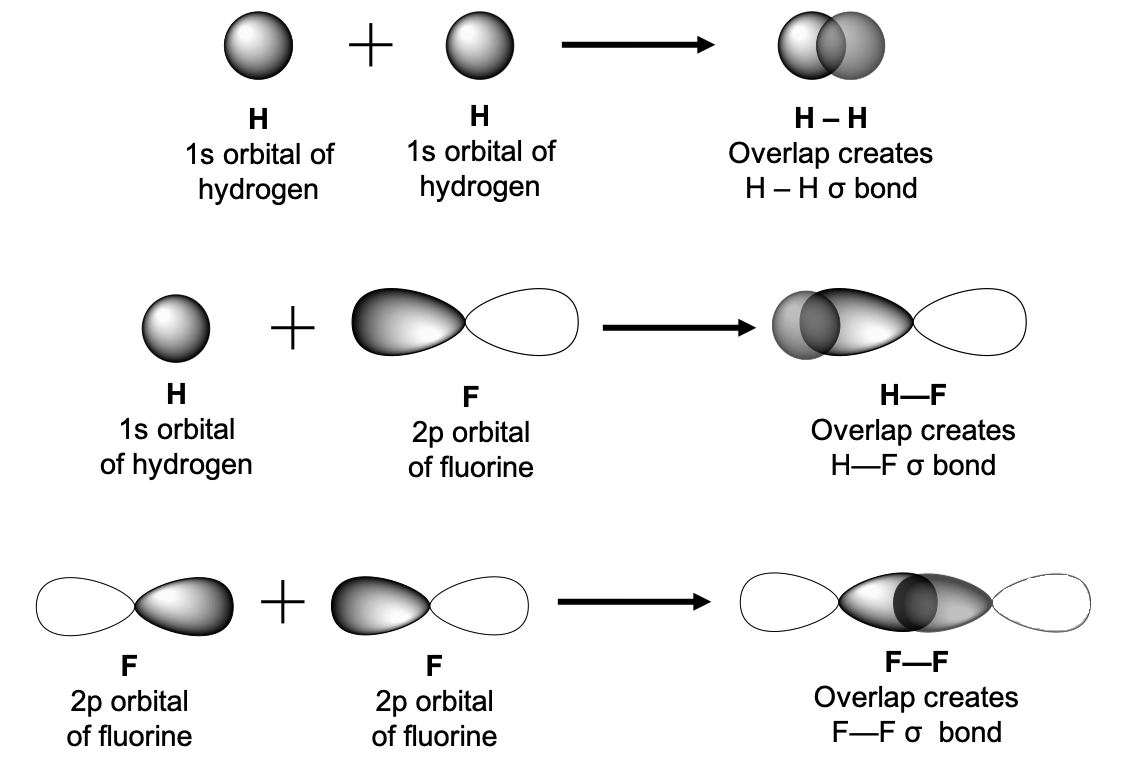Question

# What are the Applications of Valence Bond Theory?

Open in App
Solution

## Valence bond theory:In a molecule, electrons inhabit atomic orbitals instead of molecular orbitals. Because of the overlaying of atomic orbitals, a chemical bond is formed, and electrons are confined in the bond region.Applications of Valence bond theory:Valence bond theory in chemistry explores the characteristics of a chemical bond in a molecule from the perspective of atomic valencies.The rule that the central atom in a molecule seeks to establish electron-pair bonds in line with geometric restrictions specified by the octet rule is summarised by valence bond theory. The criterion of the greatest intersection is an integral feature of the Valence Bond theory since it suggests the formation of the strongest possible bonds. This concept is applied to the production of covalent bonds in a broad range of substances.Example of Applications of Valence bond theory:In the instance of the ${\mathrm{F}}_{2}$ particle, for example, the $\mathrm{F}-\mathrm{F}$ bond is produced by the crossroads of the ${\mathrm{p}}_{\mathrm{z}}$ orbitals of the two $\mathrm{F}$ atoms, each of which includes an unpaired electron. Following that, the colliding orbitals in ${\mathrm{H}}_{2}$ hydrogen and ${\mathrm{F}}_{2}$ fluorine molecules differ, as do the binding energies and bond lengths between ${\mathrm{H}}_{2}$ and ${\mathrm{F}}_{2}$ molecules.The covalent bond in a $\mathrm{HF}$ molecule is created by the intersection of the hydrogen $1\mathrm{s}$ orbital and the fluorine $2{\mathrm{p}}_{\mathrm{z}}$ orbital, both of which contain an unpaired electron.Because$\mathrm{H}$ and $\mathrm{F}$ share electrons, $\mathrm{HF}$ forms a covalent bond.Suggest Corrections3Similar questionsExplore more